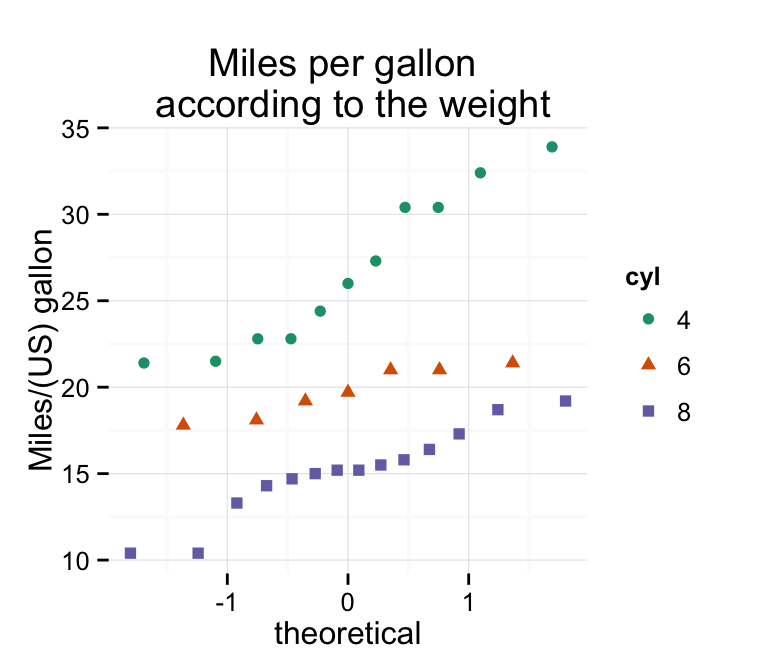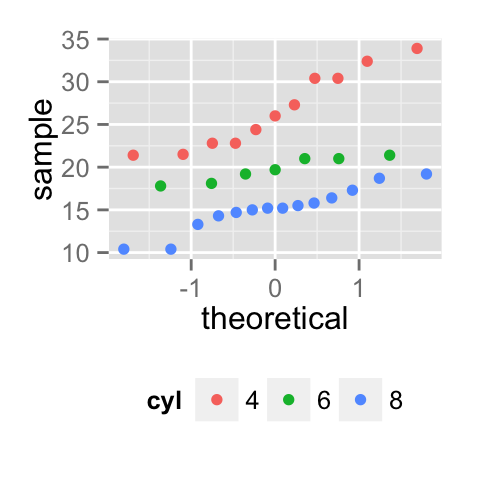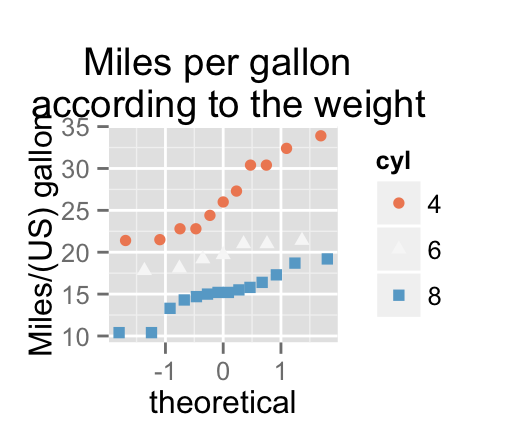# ggplot2 qq plot (quantile - quantile graph) : Quick start guide - R software and data visualization

This R tutorial describes how to create a qq plot (or quantile-quantile plot) using R software and ggplot2 package. QQ plots is used to check whether a given data follows normal distribution.

The function stat_qq() or qplot() can be used.# Prepare the data

mtcars data sets are used in the examples below.

``````# Convert cyl column from a numeric to a factor variable
mtcars\$cyl <- as.factor(mtcars\$cyl)
``````##                    mpg cyl disp  hp drat    wt  qsec vs am gear carb
## Mazda RX4         21.0   6  160 110 3.90 2.620 16.46  0  1    4    4
## Mazda RX4 Wag     21.0   6  160 110 3.90 2.875 17.02  0  1    4    4
## Datsun 710        22.8   4  108  93 3.85 2.320 18.61  1  1    4    1
## Hornet 4 Drive    21.4   6  258 110 3.08 3.215 19.44  1  0    3    1
## Hornet Sportabout 18.7   8  360 175 3.15 3.440 17.02  0  0    3    2
## Valiant           18.1   6  225 105 2.76 3.460 20.22  1  0    3    1``````

# Basic qq plots

In the example below, the distribution of the variable mpg is explored :

``````library(ggplot2)
# Solution 1
qplot(sample = mpg, data = mtcars)
# Solution 2
ggplot(mtcars, aes(sample=mpg))+stat_qq()``````# Change qq plot point shapes by groups

In the R code below, point shapes are controlled automatically by the variable cyl.

You can also set point shapes manually using the function scale_shape_manual()

``````# Change point shapes by groups
p<-qplot(sample = mpg, data = mtcars, shape=cyl)
p
# Change point shapes manually
p + scale_shape_manual(values=c(1,17,19))``````Read more on point shapes : ggplot2 point shapes

# Change qq plot colors by groups

In the R code below, point colors of the qq plot are automatically controlled by the levels of cyl :

``````# Change qq plot colors by groups
p<-qplot(sample = mpg, data = mtcars, color=cyl)
p``````It is also possible to change manually qq plot colors using the functions :

• scale_color_manual() : to use custom colors
• scale_color_brewer() : to use color palettes from RColorBrewer package
• scale_color_grey() : to use grey color palettes
``````# Use custom color palettes
p+scale_color_manual(values=c("#999999", "#E69F00", "#56B4E9"))
# Use brewer color palettes
p+scale_color_brewer(palette="Dark2")
# Use grey scale
p + scale_color_grey() + theme_classic()``````Read more on ggplot2 colors here : ggplot2 colors

# Change the legend position

``````p + theme(legend.position="top")
p + theme(legend.position="bottom")
p + theme(legend.position="none") # Remove legend``````The allowed values for the arguments legend.position are : “left”,“top”, “right”, “bottom”.

Read more on ggplot legends : ggplot2 legend

# Customized qq plots

``````# Basic qq plot
qplot(sample = mpg, data = mtcars)+
labs(title="Miles per gallon \n according to the weight",
y = "Miles/(US) gallon")+
theme_classic()
# Change color/shape by groups
p <- qplot(sample = mpg, data = mtcars, color=cyl, shape=cyl)+
labs(title="Miles per gallon \n according to the weight",
y = "Miles/(US) gallon")
p + theme_classic()``````Change colors manually :

``````# Continuous colors
p + scale_color_brewer(palette="Blues") + theme_classic()
# Discrete colors
p + scale_color_brewer(palette="Dark2") + theme_minimal()
p + scale_color_brewer(palette="RdBu")``````Read more on ggplot2 colors here : ggplot2 colors

# Infos

This analysis has been performed using R software (ver. 3.1.2) and ggplot2 (ver. 1.0.0)